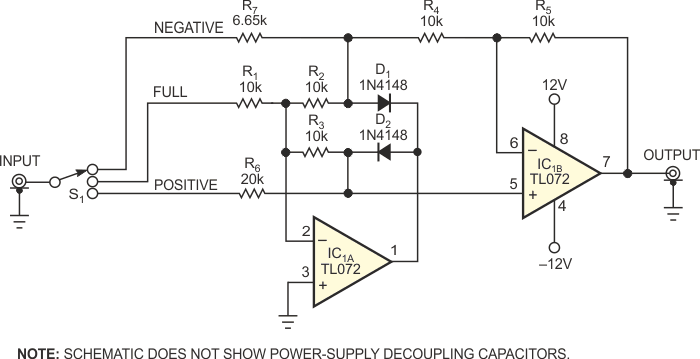# Rectifier tracks positive and negative peaks

## Texas Instruments TL072

Signals ranging from music to complex control-system waveforms may contain unequal positive and negative peak amplitudes. An "envelope-follower" circuit can track unequal peaks, but the ability to select a desired peak can enhance the circuit's performance (Reference 1). The circuit in Figure 1 applies a new twist to a classic absolute-value circuit. Applying an input signal to R1 (full) produces an output equal to the input's absolute value. Applying an input signal to R6 (positive) or R7 (negative) produces outputs of positive or negative half-cycles, respectively. Figure 2 illustrates all three modes of operation.Figure 1. Use this versatile precision rectifier circuit to recover a signal’s positive peaks, negative peaks, or both in fullwave mode.

Understanding the circuit is simple if you consider that op amp IC1A strives to maintain its inverting input at virtual ground. For example, applying –1 V to the negative input, R7, drives the anode of D1 to –333 mV. IC1A's output, Pin 1, drives D2's cathode positive enough to force D2's anode voltage to 333 mV. Because IC1A's inputs now rest at 0 V, D1 is effectively reverse-biased and out of the circuit. The 333 mV available at D2's cathode also applies to IC1B's noninverting input, Pin 5, and IC1B must balance its input voltages by driving its output, Pin 7, to 1 V. IC1B's inverting input, Pin 6, goes to 333 mV. The voltage drop across R4 thus equals 666 mV. One-third of the input current flows through the series connection of R2 and R3, and two-thirds flows in R4. To achieve unity gain, R7's value equals that of R2 + R3 in parallel with R4.Figure 2. This waveform plot shows the circuit’s outputs for a sine-wave input connected to the negative, full, and positive inputs, respectively. Traces are vertically offset for clarity.

Applying a positive input to R7 causes IC1A's output to go negative by a voltage equal to one forward-diode drop and thus holds D1's anode at ground. D2 is reverse-biased, and both of IC1B's inputs rest at 0 V. The circuit's output is thus 0 V. Applying an input voltage at R6 yields similar operation. A positive input causes an equal-value positive output, and a negative input produces a 0 V output. You can ignore the effects of IC1B's high input impedance, which are negligible. To maintain unity gain, the value of R6 is twice that of R3.

Resistors R1, R2, R3, R4, and R5 are of equal value and close tolerance. Note that IC1's power-supply connections require bypass capacitors (not shown). To minimize errors, use a low-impedance source or buffer amplifier to drive the circuit. You can use a three-position rotary switch for input-mode selection, or an on/on/on toggle switch, such as C&K Components' 7211 or a similar switch, wired as a three-way selector. (See the manufacturer's data sheet for a connection diagram.) You can also use separate connectors for the inputs, but connect no more than one input at a time.

EDN